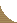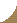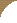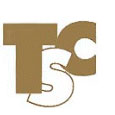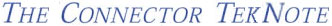May 2001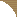This is the second of a series of quarterly reviews on selected topics of electrical/electronic connector technology. You are invited to suggest a review topic of interest.

Roland S. Timsit, President
Timron Scientific Consulting Inc.
Tel: 416-787-8161
, www.timron-inc.comThe Temperature of an Electrically Heated Contact Spot

In designing reliable electronic and electrical connectors, it is essential that a minimum specification on contact resistance be met. One of the drivers for this specification is the requirement that contact temperature does not exceed a maximum value under the worst-case electrical loading condition. An unacceptably elevated contact temperature leads to accelerated connector degradation. This degradation stems from the action of mechanisms such as loss of mechanical contact load due to stress relaxation and metal flow, oxidation, interdiffusion and intermetallics formation etc [1,2]. In this newsletter, we review the contact parameters that determine contact temperature during passage of an electrical current.

Contact Resistance and Contact temperature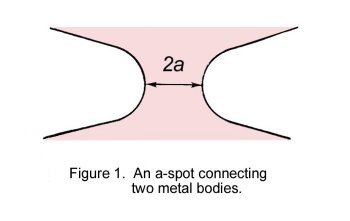The electrical resistance of two bodies of electrical resistivity ρ joined by a circular constriction of radius a, as shown in Fig.1, is given as ρ/2a [1,3]. If a DC electrical current I passing through the contact spot (also known as a-spot ) does not heat the a-spot significantly, the voltage drop across the constriction is simply given as ρI/2a. Under conditions where significant joule heat is produced within the constriction, the crowding of current lines within the a-spot generates a thermal gradient in the vicinity of that constriction. The steady-state thermal gradient produced by joule heating of a circular a-spot linking two identical metallic bodies is shown schematically in Fig. 2 . The maximum temperature Tm occurs in the plane of the constriction. The effect of the temperature gradient complicates the relationship between voltage-drop across the contact, the electrical current and the a-spot dimension. We now examine the nature of this relationship.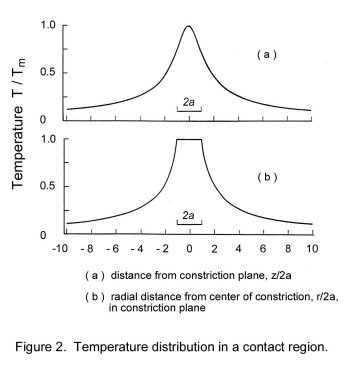The simplest way of understanding the relationship of contact temperature and joule heating in the contact region is to consider the steady-state temperature produced within an electrically conductive bridge connecting two metal slabs. This is illustrated in Fig.3. We assume that the length of the conducting bridge is 2L and the rectangular cross section is A. We also assume that the temperature of the two metals slabs is uniform and given as To. An electrical current of uniform density j is passed through the bridge, also as shown in Fig. 4. If all conductors are thermally insulated from the surroundings, the differential equation governing the temperature distribution T(x) along the metal bridge is 

 λd2T(x)/dx2 = - ρj2 (1)

where λ and ρ are the thermal conductivity and electrical resistivity of the conductor material respectively, and x is the distance along the conductor with x=0 taken at the center of the bridge. Equation (1) states that the joule heat ρj2 generated per unit volume within the connecting bridge is dissipated by thermal conduction in the metal. If for simplicity λ and ρ are assumed temperature independent, the solution of Eq. (1) is straightforward and is given as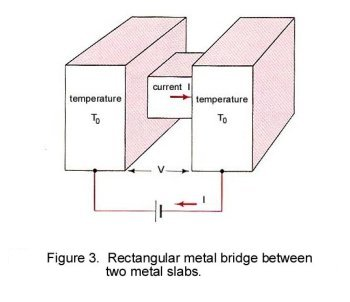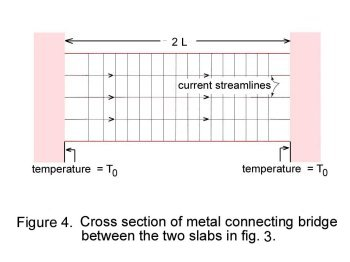T(x) = To + (ρ/λ) j2 ( L2 - x2 ). (2)

Note that the temperature distribution within the conductor is parabolic with the maximum T(0) occurring at the center x = 0 of the conductor. The minimum temperature To occurs at x = L and x = - L. We shall designate the maximum temperature T(0) as Tm. Since λ and ρ are assumed to be temperature independent, the voltage drop across the bar is given as

 V = 2ρLI / A = 2ρjL

where j is the current density given as I/A. The difference between the maximum temperature in the bridge and the temperature To at the ends i.e. the Supertemperature, is given as

 Tm - To = V2/8ρλ (3)

Note the following important points in Eq.(3), also known as the Voltage-Temperature relation:
1. the Supertemperature [ Tm - To ] is determined by the voltage-drop across the metal bridge,
2. although the temperature distribution within the connecting bridge depends on the bridge geometry as indicated in Eq.(2), the Supertemperature described by Eq.(3) does not depend explicitly on contact geometry,
3. Eq.(3) is valid only where heat is dissipated from the conductor only by conduction to the ends of the conductor and to the two metal slabs held at the temperature To. No heat is lost to the surrounding environment (i.e. air) from the conductor sides since the conductor is thermally insulated.
The absence of dependence of the Supertemperature on conductor geometry indicates that the relationship expressed by Eq. (3) holds for an electrical junction of any shape including the shape shown in Fig. 5. In that junction, the temperature distribution follows the pattern illustrated in Fig. 2 and drops to the value To of the bulk contacting bodies within a short distance from the constriction. Again, we remind the reader that the temperature distribution within the junction in Fig.5 is not parabolic as indicated in Eq.(2), since the temperature distribution depends on contact geometry. Only the Supertemperature [ Tm - To ] is independent of contact geometry.

The Voltage-Temperature (V-T) relation expressed in Eq.(3) is a special case of the general relation between voltage and maximum temperature [3,4] in a thermally insulated body carrying an electrical current, given as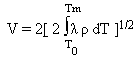(4)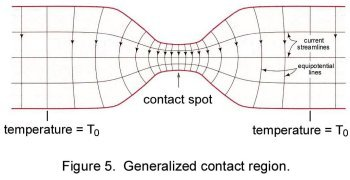In the above expression, the parameters λ and ρ may be temperature dependent. It may be easily verified that Eq.(4) reduces to Eq.(3) where λ and ρ are constant (i.e. do not vary with temperature). The general V-T relation expressed by Eq.(4) identifies the voltage-drop across a contact, not the electrical current, as the fundamental parameter for contact temperature.

The Wiedemann-Franz Law

For many connector materials, there is relationship linking the electrical resistivity ρ and the thermal conductivity λ. This relationship is known as the Wiedemann-Franz law and states that the product λρ depends only the absolute temperature, as

 λρ = LT (5)

where L is the Lorenz constant (2.45 x 10-8 (V/K)2) and T is the absolute temperature (in kelvin). Although this relation provides a reasonable description of thermal and electrical transport properties in metals over the temperature range generally relevant to ordinary electrical contacts, it is not universally valid. The origin of the Wiedemann-Franz law is discussed in reference . If the relation (5) holds, then Eq.(4) immediately yields the rigorously valid V-T relation as

 V2 = 4L ( Tm2 - To2 ) (6)

which is independent of contact geometry and of the materials in contact. It may easily be shown that Eq.(6) reduces to Eq.(3) when the thermal conductivity and the electrical resistivity are independent of temperature . The dependence of the maximum contact temperature Tm on the voltage V across a constriction is shown in Fig. 6. The contact temperatures were evaluated from Eq.(6) for a bulk temperature To of 20oC and 100oC. Note that a voltage-drop of 0.3 to 0.4 volt leads to contact temperatures that exceed the melting point of most electrical contact materials. The "softening" and "melting" voltages for several contact materials are listed in Table 1.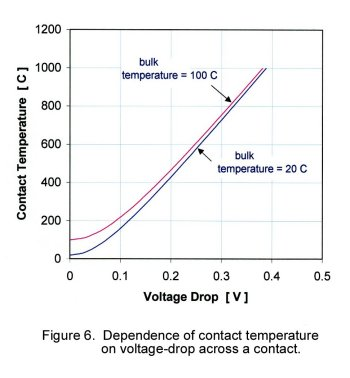Table 1
Voltage for Softening (Vs) and Melting (Vm)
of Common Electrical Contact Materials

 Material VsSoftening(V) VmMelting(V) AlFeNiCuZnMoAgCdSnAuWPtPdPb60Cu-40Zn60Cu-40SnStainless SteelWC, Co 0.10.190.160.120.10.250.090.070.080.40.250.120.270.6 0.30.190.160.430.170.750.370.150.130.431.10.650.570.190.20.150.55

Thermal Risetime of an Electrically Heated Contact Spot

The above discussion addressed the V-T relation in contacts heated by a DC current where a thermal steady state condition had been reached. What happens when the electrical is alternating and contact heating varies with time? Fortunately, the description of contact temperature in terms of voltage-drop remains simple. It has been shown [1,3] that the time constant that characterizes temperature rise in the neighbourhood of a circular constriction of radius a, due to joule heating, is ca2/4λ where c is the heat capacity and λ is the thermal conductivity . For electrical conductors such as copper, λ and c take values of 400 W m-1 oC-1 and 3.44x106 J m-3 respectively. The time constant is thus 2.2x10-7 second for a constriction of radius of 10 μm in a copper-copper interface. The small risetime associated with the heating of an a-spot forms the basis on which thermal transients are usually ignored in dealing with stationary or slowly moving contacts. In these applications, only the equilibrium contact temperature is of interest. Thermal transients are taken into account only in applications of rapidly moving contacts such as brush contacts, or in high frequency/high-power electrical connections.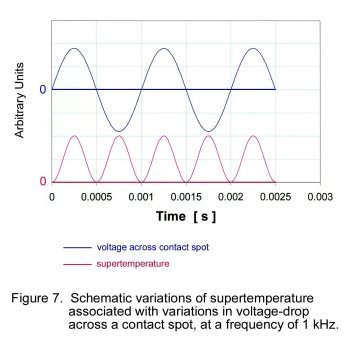Due to the small thermal risetime associated with a-spot heating, the contact temperature follows voltage variations across a contact almost instantaneously, so long as the frequency of the applied voltage does not exceed about 100 kHz. Figure 7 shows schematically the variations in contact temperature associated with sinusoidal variations in voltage-drop across the contact at a frequency of 1 kHz, when the temperature swing is not excessive. Note that temperature variations are also sinusoidal and occur at a frequency of 2 kHz. This doubling of temperature fluctuation frequency stems from the dependence of a-spot temperature on the square of the voltage V. The temperature fluctuations will be distorted from purely sinusoidal when the maximum temperature approaches the softening point of the contact materials.

Summary

The parameter that relates directly to the temperature in an a-spot is the voltage-drop across the contact, not the current. Temperature variations due to alternating voltage across the contact occur at twice the frequency of the applied voltage.

References
1. Timsit, R.S., "Electrical Contact Resistance: Fundamental Principles", in Electrical Contacts, Principles and Applications, Ed. Slade, P.G., p. 1, Marcel Dekker, Inc., New York, 1999.
2. Mroczkowski, R.S., Electric Connector Handbook, McGraw-Hill, New York, 1998.
3. Holm, R., Electric Contacts, Theory and Applications, Springer-Verlag, Berlin, 1976.
4. Greenwood, J.A. and Williamson, J.B.P., "Electrical Conduction in Solids, II. Theory of Temperature-Dependent Conductors," Proc. Roy. Soc., A246, p. 13 1958.
5. Carlslaw, H.S. and Jaeger, J.C., Conduction of Heat in Solids, Oxford University Press, London, 1959.Copyright ©2005 Timron Scientific Consulting Inc. 467 Woburn Ave, Toronto, Ontario, Canada M5M 1L6. Phone : 416-787-8161, Fax : 416-787-4660.